# Root to Leaf Path having sum X in the given Tree

In this article, we are going to see how to find whether there is any root to leaf path having sum X in the given tree?
Submitted by Radib Kar, on September 10, 2020

Here, we are going to see how we can find whether the given tree has any root to leaf path having sum X?

In the below example,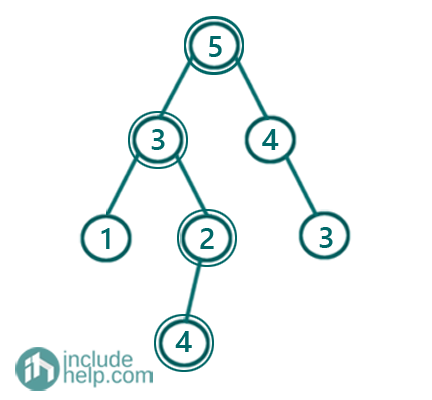In the above given tree, if sum X is 14, then we find a root to leaf path which is marked in the above tree ( 5->3->2->4)

But, if sum X is 13, then we don't find any root to leaf path having the sum.

Solution:

We can solve this recursively like the below algorithm:

We keep calculating sum from the root and if reaching any node the cumulative sum becomes X, then we find a path having sum X.

Initially, cur_sum is 0 which stores cumulative sum for paths.

```Function hasPathSumRecur(node, sum X , cur_sum):
If root is NULL
return FALSE;
Add current node value to cur_sum
If current node is a leaf node & && cur_sum  equals to X
return TRUE as we find a path;
Otherwise recur for both the subtrees and
if we find any one satisfying then return TRUE else FALSE
```

C++ Implementation:

```#include <bits/stdc++.h>
using namespace std;

// tree node is defined
class TreeNode {
public:
int val;
TreeNode* left;
TreeNode* right;
TreeNode(int data)
{
val = data;
left = NULL;
right = NULL;
}
};

bool hasPathSumRecur(TreeNode* root, int sum, int cur_sum)
{
if (!root)
return false;
cur_sum += root->val;
if (!root->left && !root->right && cur_sum == sum)
return true;
return hasPathSumRecur(root->left, sum, cur_sum) || hasPathSumRecur(root->right, sum, cur_sum);
}

bool hasPathSum(TreeNode* root, int sum)
{
int cur_sum = 0;
return hasPathSumRecur(root, sum, cur_sum);
}

int main()
{
//building the tree like the example
TreeNode* root = new TreeNode(5);

root->left = new TreeNode(3);
root->right = new TreeNode(4);
root->left->left = new TreeNode(1);
root->left->right = new TreeNode(2);
root->right->right = new TreeNode(3);
root->left->right->left = new TreeNode(4);

int sum = 14;

if (hasPathSum(root, sum))
cout << "There is a root to leaf path having sum: " << sum << endl;
else
cout << "There is no root to leaf path having sum: " << sum << endl;

sum = 13;

if (hasPathSum(root, sum))
cout << "There is a root to leaf path having sum: " << sum << endl;
else
cout << "There is no root to leaf path having sum " << sum << endl;

return 0;
}
```

Output:

```There is a root to leaf path having sum: 14
There is no root to leaf path having sum 13
```

Dry run:

Initially, cur_sum is 0, X=14 and given tree is the same as the example.

Below are the steps for a dry run: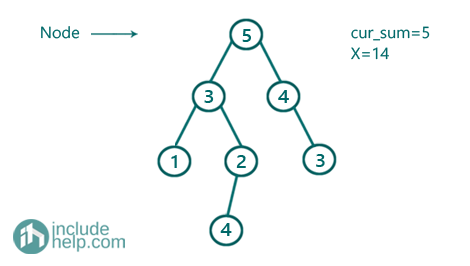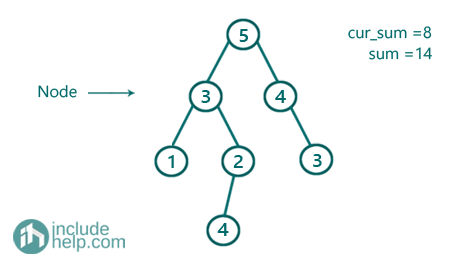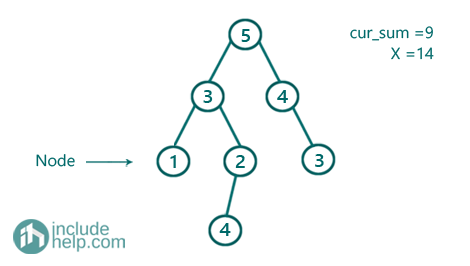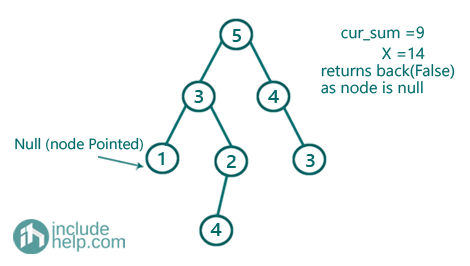Now it recurs for the right child of 1 which is again NULL and thus controls returns back to node 3. And now there we recur for the right subtree of 3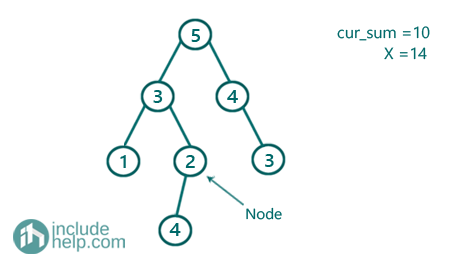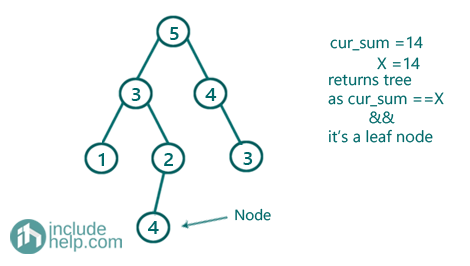So, we find a path here and it returns true.

You can do the dry run taking X=13 and you will find that no path has the sum 13.

What's New

Top Interview Coding Problems/Challenges!

Languages: » C » C++ » C++ STL » Java » Data Structure » C#.Net » Android » Kotlin » SQL
Web Technologies: » PHP » Python » JavaScript » CSS » Ajax » Node.js » Web programming/HTML
Solved programs: » C » C++ » DS » Java » C#
Aptitude que. & ans.: » C » C++ » Java » DBMS
Interview que. & ans.: » C » Embedded C » Java » SEO » HR
CS Subjects: » CS Basics » O.S. » Networks » DBMS » Embedded Systems » Cloud Computing
» Machine learning » CS Organizations » Linux » DOS
More: » Articles » Puzzles » News/Updates